# Texas Go Math Grade 5 Lesson 15.1 Answer Key Make Frequency Tables

Refer to our Texas Go Math Grade 5 Answer Key Pdf to score good marks in the exams. Test yourself by practicing the problems from Texas Go Math Grade 5 Lesson 15.1 Answer Key Make Frequency Tables.

## Texas Go Math Grade 5 Lesson 15.1 Answer Key Make Frequency Tables

Frequency is the number of times an event occurs. A frequency table is a table that uses numbers to record data about how often something happens.

Unlock the Problem

Bart kept a record of the sizes of bags of peanuts he sold at a baseball game.Make a frequency table of the data.
Step 1: Write the title at the top of the frequency table.
Step 2: List the sizes of bags of peanuts in the first column.
Step 3: Record the frequency of each size of bag of peanuts sold in the frequency column.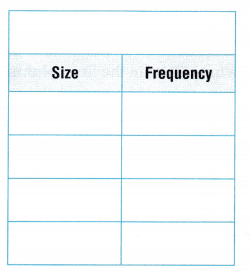• If you want to know quickly how many large bags of peanuts Ban sold, would you use his record or would you use the frequency table? Explain.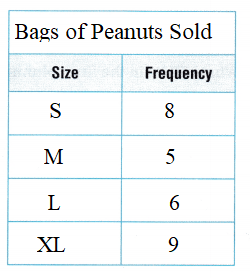Use the frequency table with Bart’s record, I need to count the number of large bags with the frequency table, I just have to read the number.

Try This! Make a frequency table.

A librarian records the types of books students checked out one morning. Make a frequency table of the data.

• Write a title for the frequency table.
• List the types of books in the first column.
• Record the frequency of each type of book in the second column.Share and Show

Len records the methods of transportation his classmates take to school. Use the data for 1-2.

Question 1.
What is a good title for a frequency table of the data?
Transportation to school
What will be in the first column of the frequency table?
Methods of Transportation
What will be in the second column of the frequency table?
FrequencyQuestion 2.
Make a frequency table.
Bus: 9
Car: 5
Walk: 7

Math Talk
Mathematical Processes
Explain how you found the frequency of each method of transportation.

Problem Solving

A swimming coach records the events swimmers on a team are competing in. Use the data for 3-4.

Question 3.
Make a frequency table of the data. Explain how you determine what to put in the first column of the table.
Look at chart-frequencies are freestyle, backstroke, breast stoke and butterfly.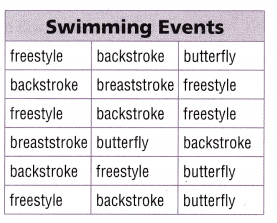Question 4.
Multi-Step How can you prove that the total number of frequencies in your table is correct?
Count the total number of events recorded. It is 18. Compare 18 to the sum of the numbers in my frequency column
6 + 6 + 2 + 4 = 18

Problem Solving

A restaurant owner recorded salsa flavors that customers asked for at dinner. Use the data for 5-7.Question 5.
Representations Make a frequency table of the data.
Frequencies are spicy 6, chunky 4, mild 3, hot 7.

Question 6.
H.O.T. Describe how the frequency table will change if 4 customers switch from chunky salsa to spicy salsa and 3 customers switch from mild salsa to hot salsa.
The table will not include chunky or mild salsa data. Spicy and hot increase to 10.

Question 7.
Multi-Step Think of a topic for a frequency table. Record a set of data for the topic. Use the data to make a
frequency table.Daily Assessment Practice

Question 8.
Marsha records the breeds of dogs in a dog show. Then she makes a frequency table to show the data. How many different breeds of dogs will be in the frequency table?(A) 2
(B) 12
(C) 3
(D) 4

Students place an order for different sizes of school sweatshirts. They make a frequency table to show the data. Use the frequency table for 9-10.

Question 9.
Students order sizes large, small, large, large, medium, small, large, small, large, medium, and small. What frequency should be in the row for medium in the frequency table?(A) 5
(B) 2
(C) 4
(D) 3
Multi-Step Students pay $14 for size small sweatshirts and$16 for size large. How much do they pay for the size small and large sweatshirts they order?
(A) $56 (B)$136
(C) $80 (D)$30
Answer: (B) $136 Texas Test Prep Question 11. Paula recorded the colors of all her shoes.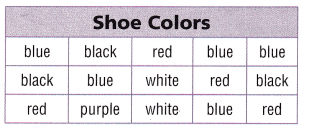She makes a frequency table of the data. What is the frequency of red shoes? (A) 3 (B) 4 (C) 15 (D) 2 Answer: (B) 4 ### Texas Go Math Grade 5 Lesson 15.1 Homework and Practice Answer Key Patricia records the types of penguins she sees in the Antarctic exhibit at the zoo. Use the data for 1-2. Question 1. What is a good title for a frequency table of the data? Answer: Antarctic Penguins What will be in the first column of the frequency table? Answer: Names of Antarctic Penguins What will be in the second column of the frequency table? Answer: FrequencyQuestion 2. Complete the frequency table at the right.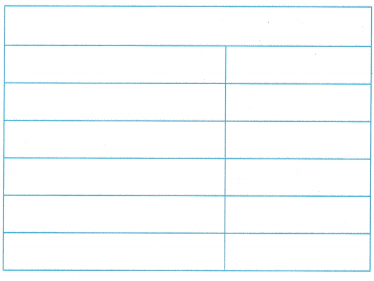Answer: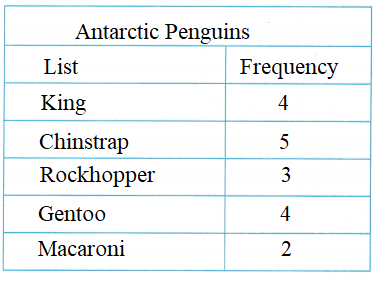Problem Solving Mr. Rexford’S students recorded the polygons in a sculpture at the modern art museum. Use the data for 3-4. Question 3. Complete the frequency table of the data.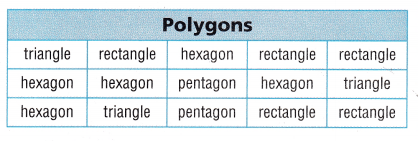Answer: triangle 3 hexagon 5 rectangle 5 pentagon 2 Question 4. Which polygon had the greatest frequency? Which polygon had the least frequency?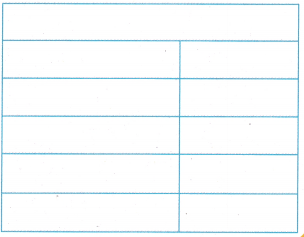Answer: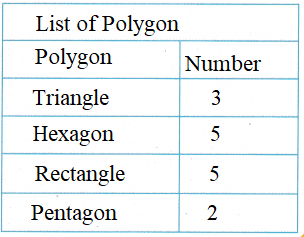Texas Test Prep Lesson Check Fill in the bubble completely to show your answer. Question 5. Milo records the types of shells he collects on the Texas coast. Then he makes a frequency table to show the data. How many different types of shells will be in the frequency table? (A) 20 (B) 4 (C) 5 (D) 3Answer: (C) 5 There are 5 different types of shells they are clam, cockle, scallop, cockle, whelk. Question 6. Carmen recorded the types of vehicles she saw while looking out the window of her father’s car. She makes a frequency table of the data. What is the frequency of motorcycles? (A) 5 (B) 6 (C) 7 (D) 3Answer: (B) 6 Josef has a pottery booth at the craft fair. He makes a frequency table to record the types of pottery sold one day. Use the frequency table for 7-8.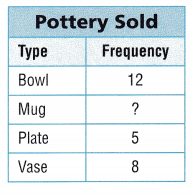Question 7. Multi-Step The number of mugs Josef sold is one fourth of the combined number of bowls and vases sold. What frequency should be in the row for mug in the frequency table? (A) 3 (B) 5 (C) 2 (D) 80 Answer: 12 + 8 = 20 20/4 = 5 Thus the correct answer is option B. Question 8. Multi-Step The price of a plate is$6 and the price of a mug is $5. How much did Josef collect from the plates and the mugs he sold? (A)$55
(B) $25 (C)$30
(D) $11 Answer: (C)$30